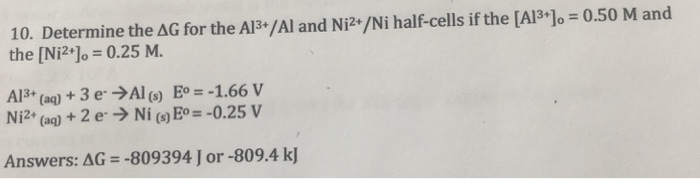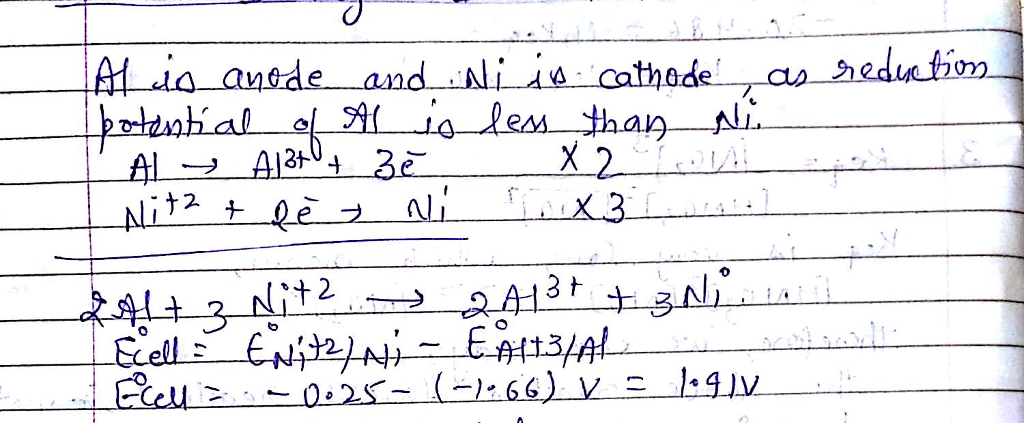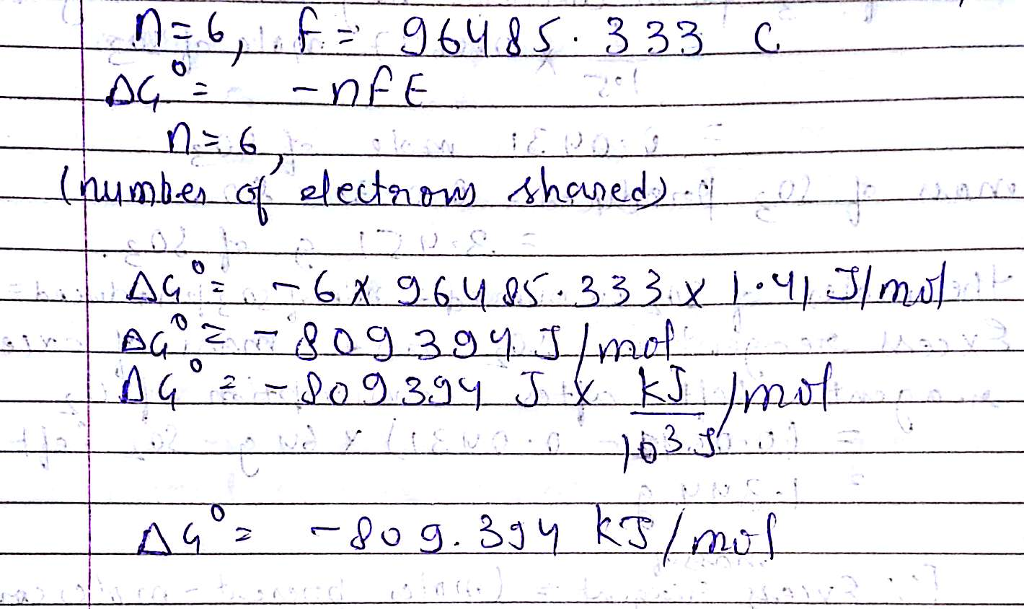# Question & Answer: Determine the delta G for the A1^3+/Al and Ni^2+/Ni half-cells if the [Al^3+]_0 = 0.50 M…..Determine the delta G for the A1^3+/Al and Ni^2+/Ni half-cells if the [Al^3+]_0 = 0.50 M and the [Ni^2+]_0 = 0.25 M. Al^3+ (aq) + 3 e^- rightarrow Almostequalto_(s) E degree = -1.66 V Ni^2+ (aq) + 2 e^- rightarrow Ni_(s) E degree = -0.25 V delta G =-809394 J or -809.4 kJ